# RS Aggarwal Solutions for Class 7 Maths Exercise 7A Chapter 7 Linear Equations in One Variable

RS Aggarwal Solutions for Class 7 Maths Exercise 7A Chapter 7 Linear Equations in One Variable, an equation is a statement of equality which contains one or more unknown quantities or variables. An equation involving only a linear polynomial is a linear equation. Students of Class 7 are suggested to solve RS Aggarwal Class 7 Solutions Chapter 7 to strengthen the fundamentals and be able to solve questions that are usually asked in the examination. By practising RS Aggarwal Solutions for Class 7 Maths Chapter 7 Linear Equations in One Variable, students can get good marks in Maths.

## Download the PDF of RS Aggarwal Solutions For Class 7 Maths Chapter 7 Linear Equations in One Variable – Exercise 7A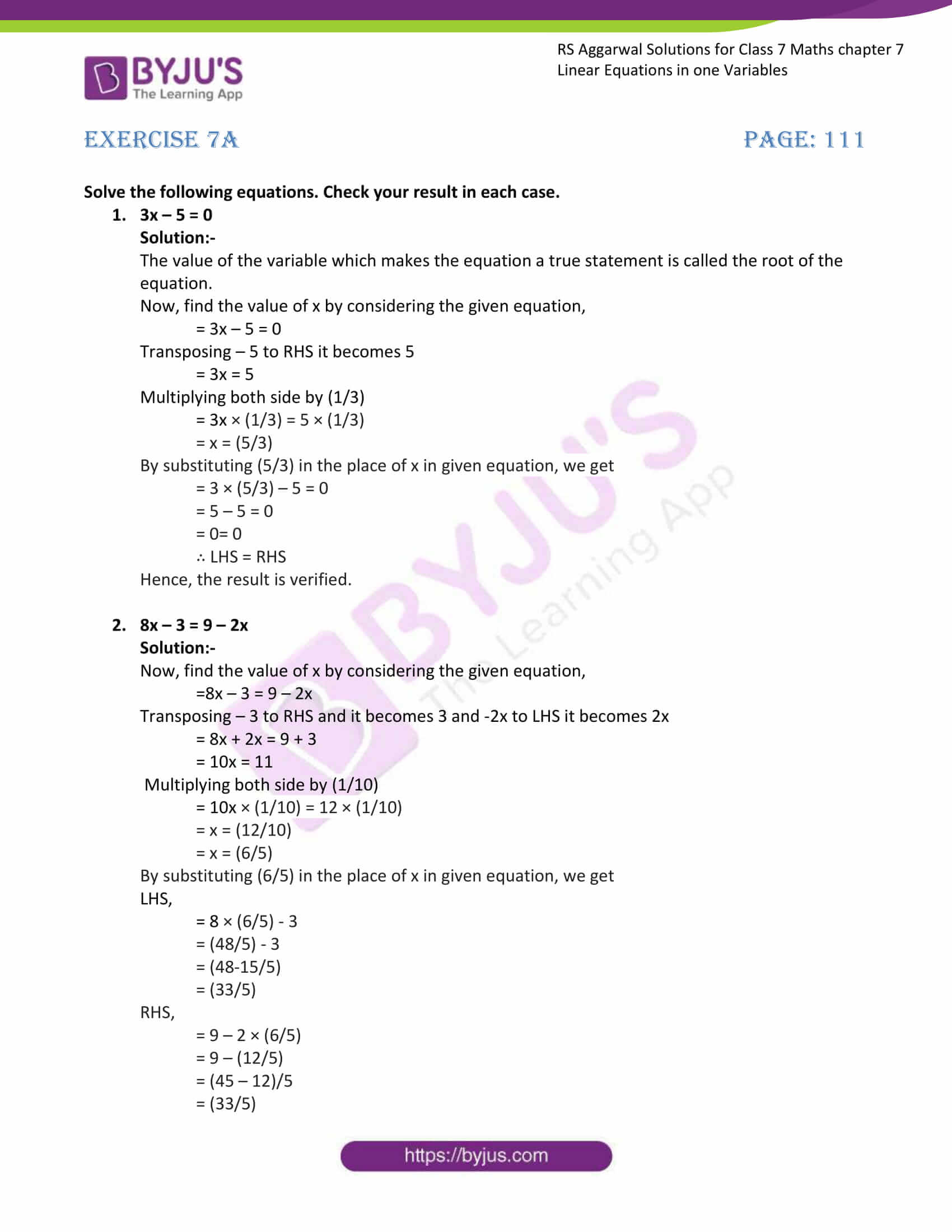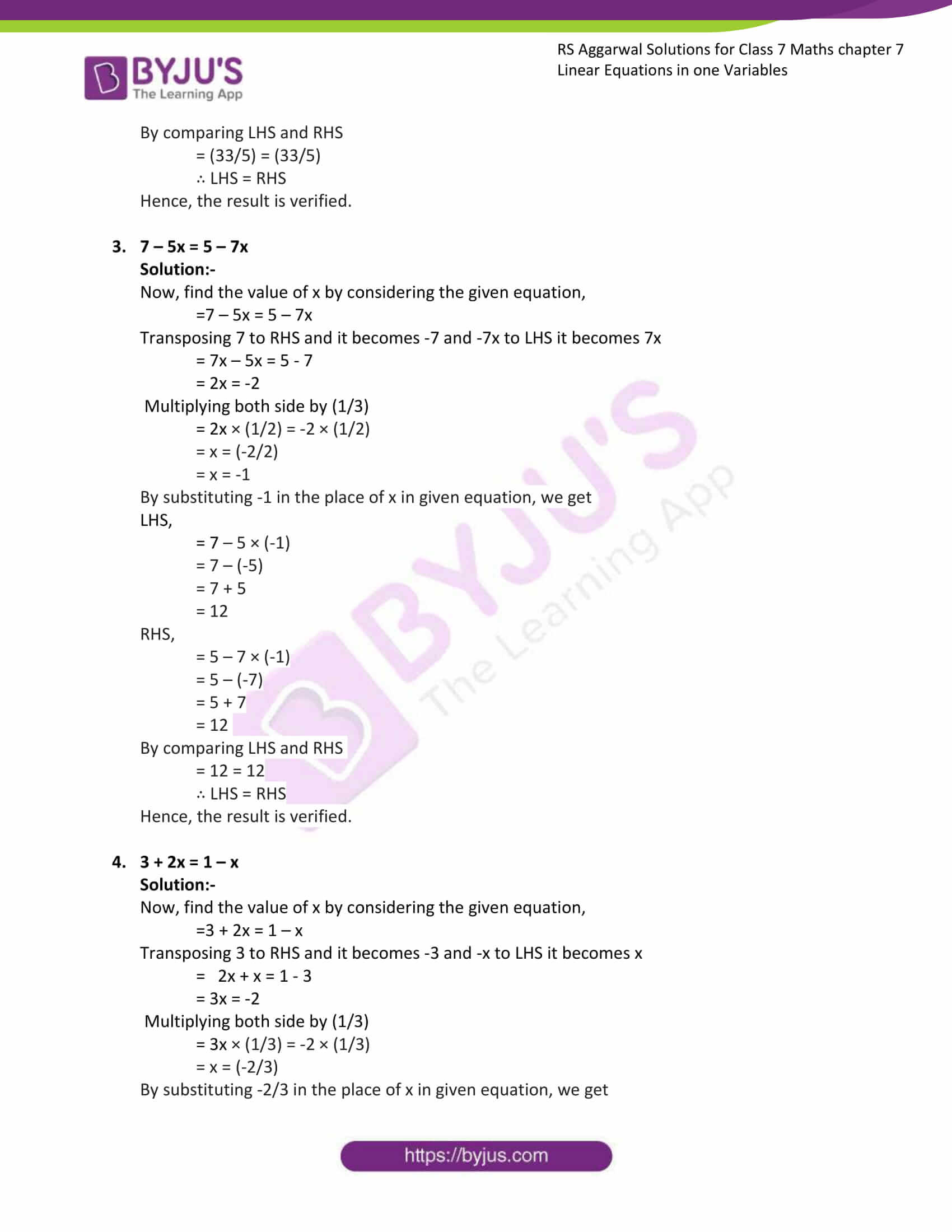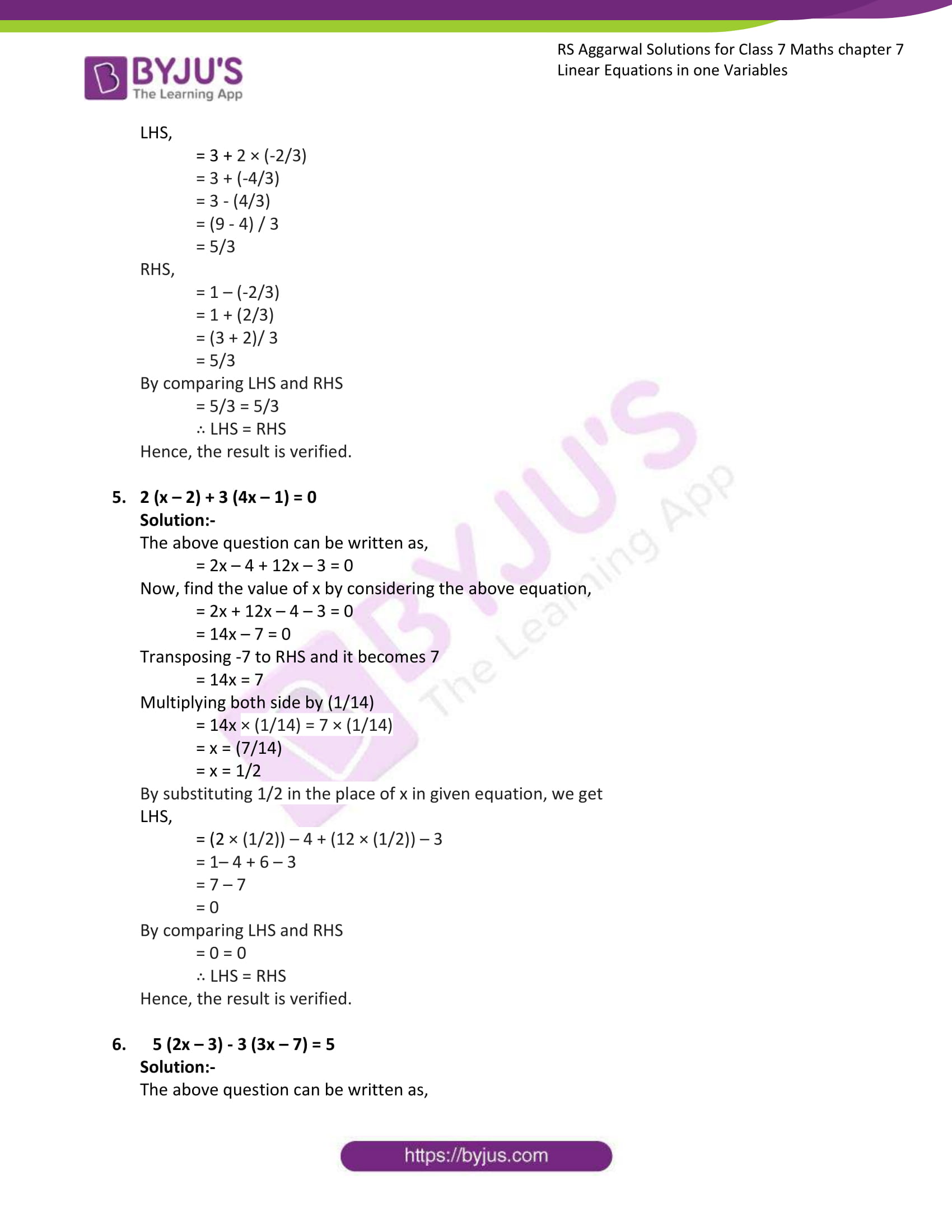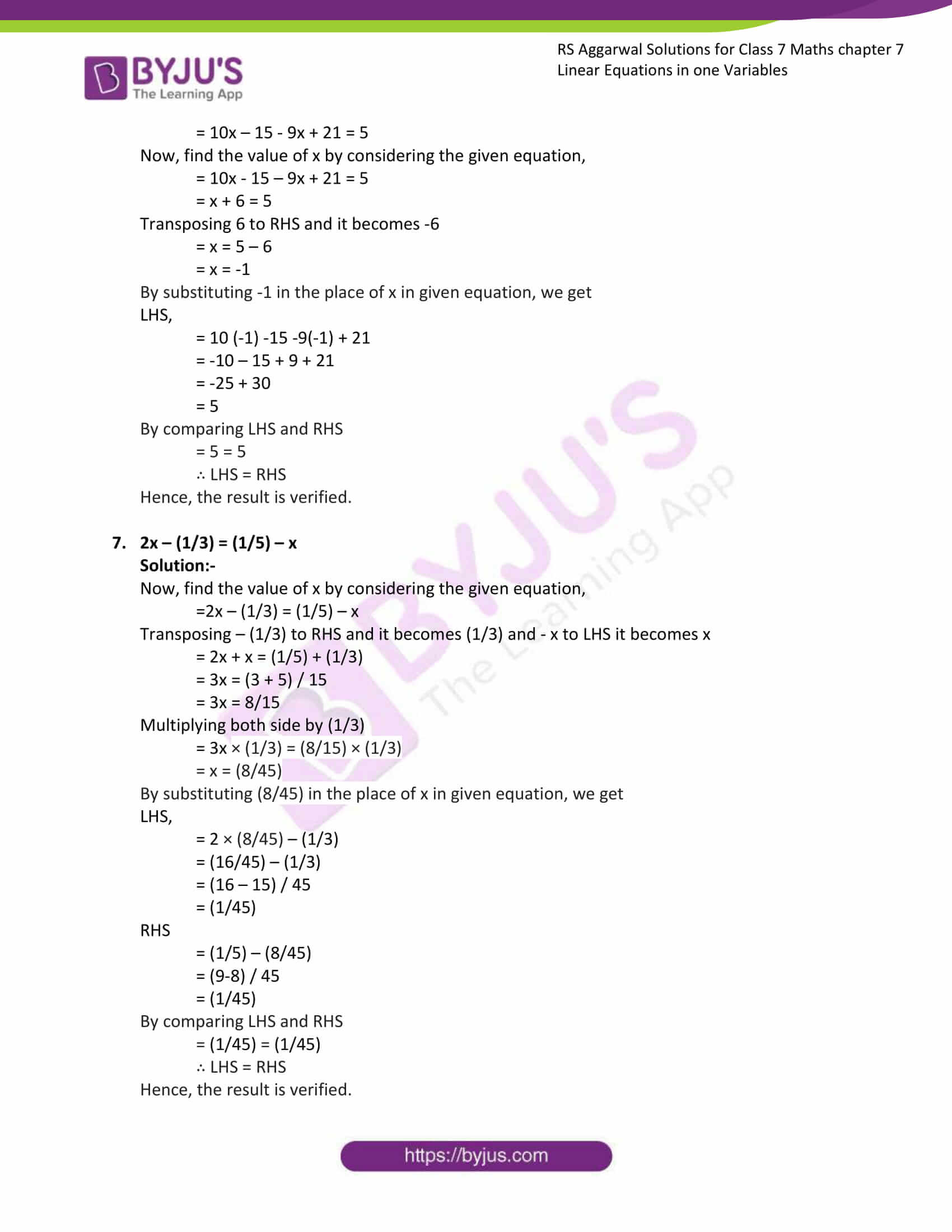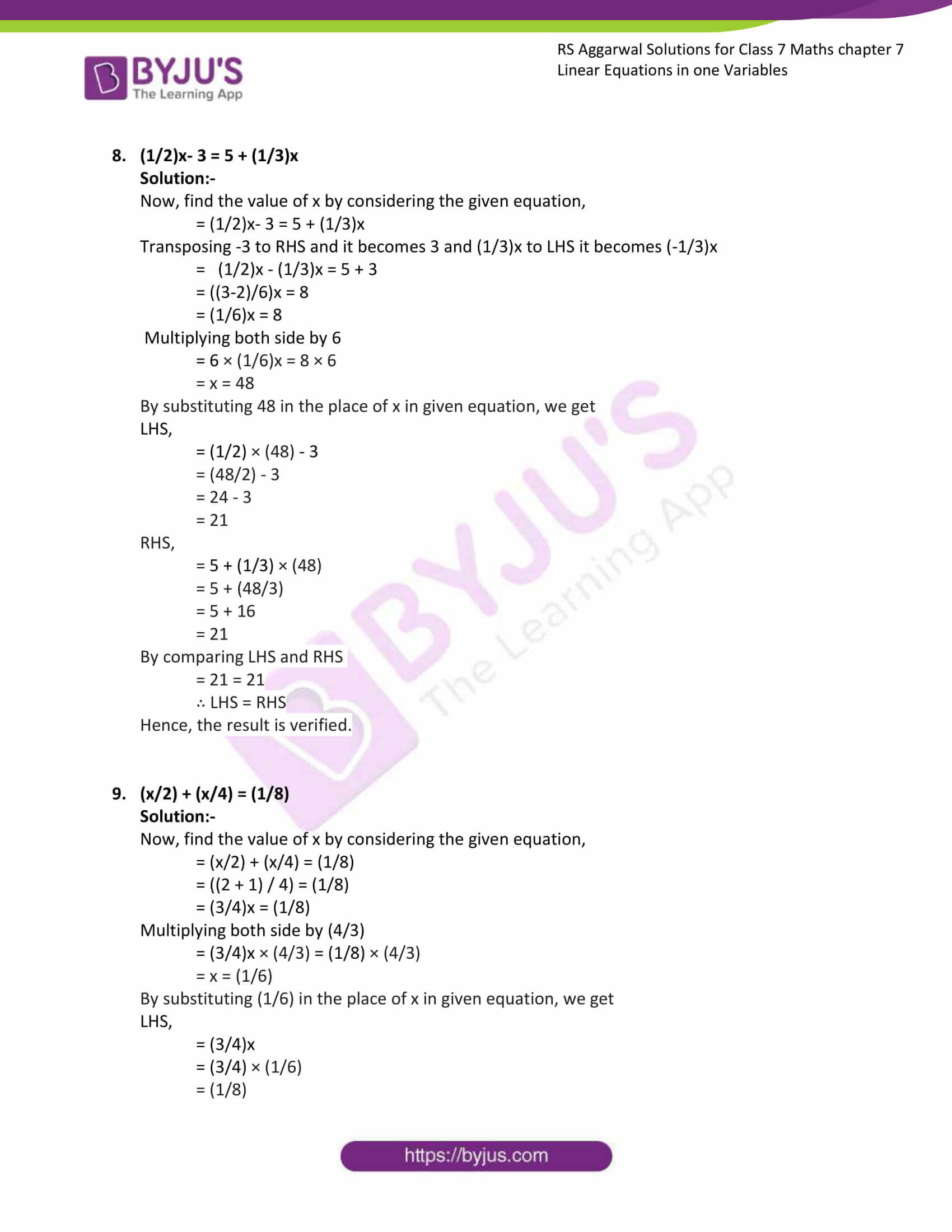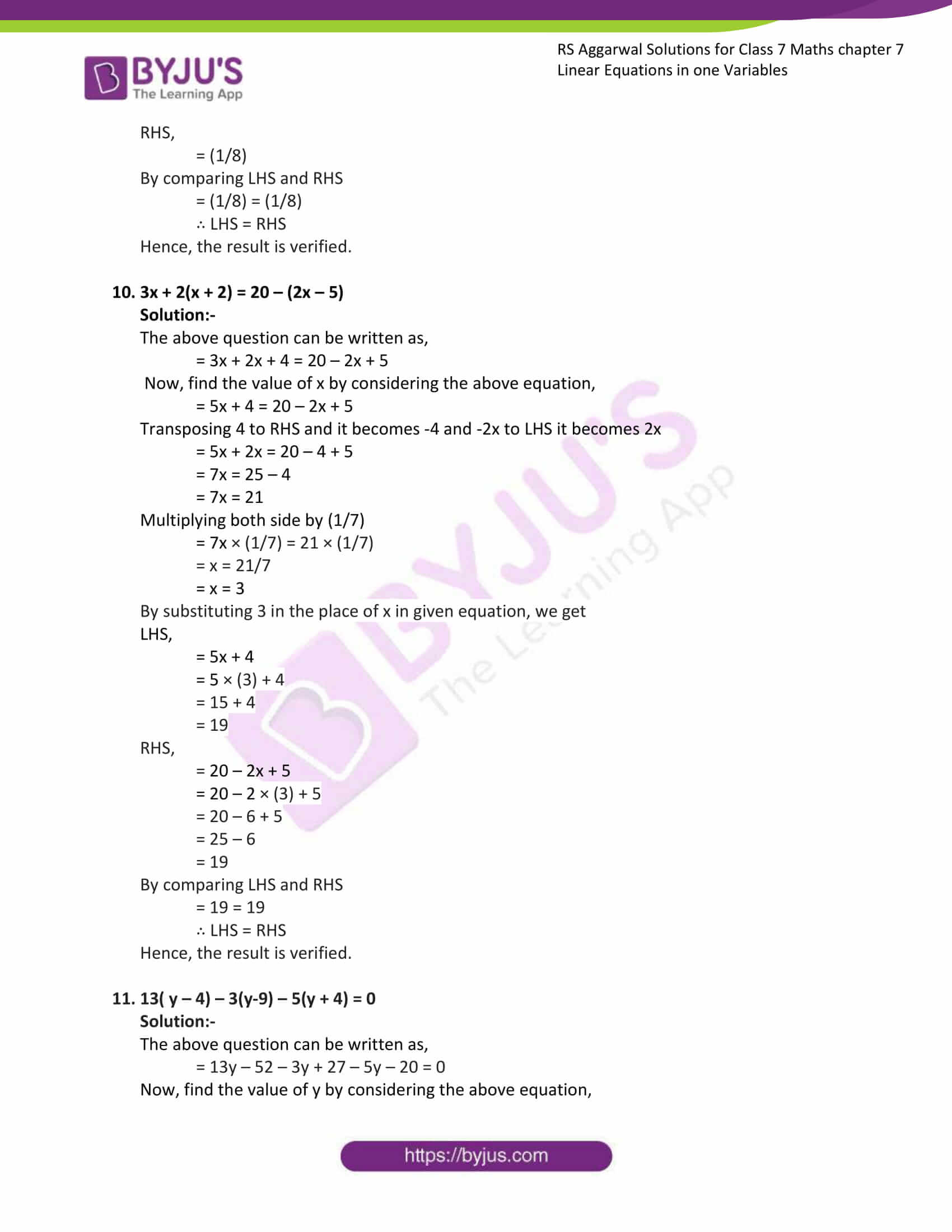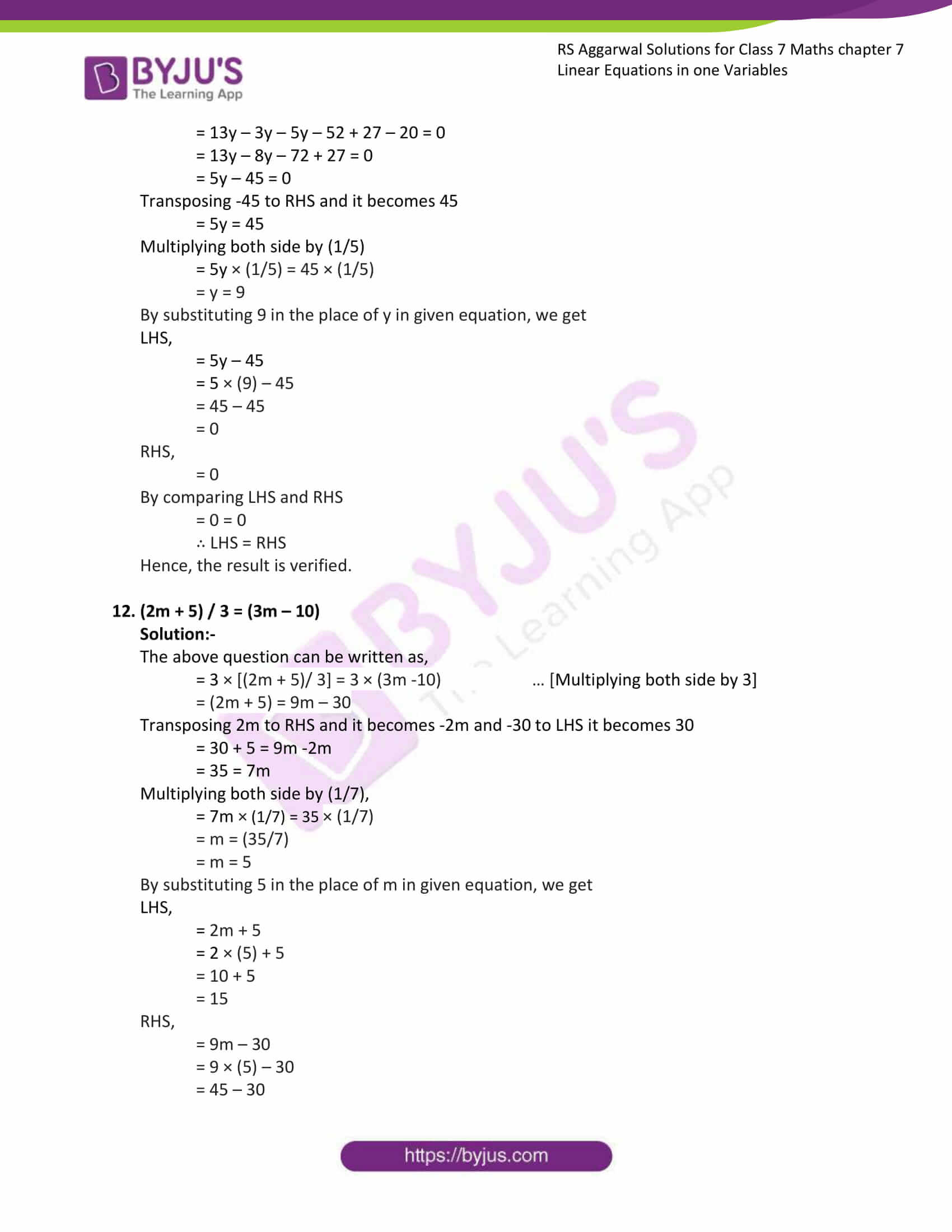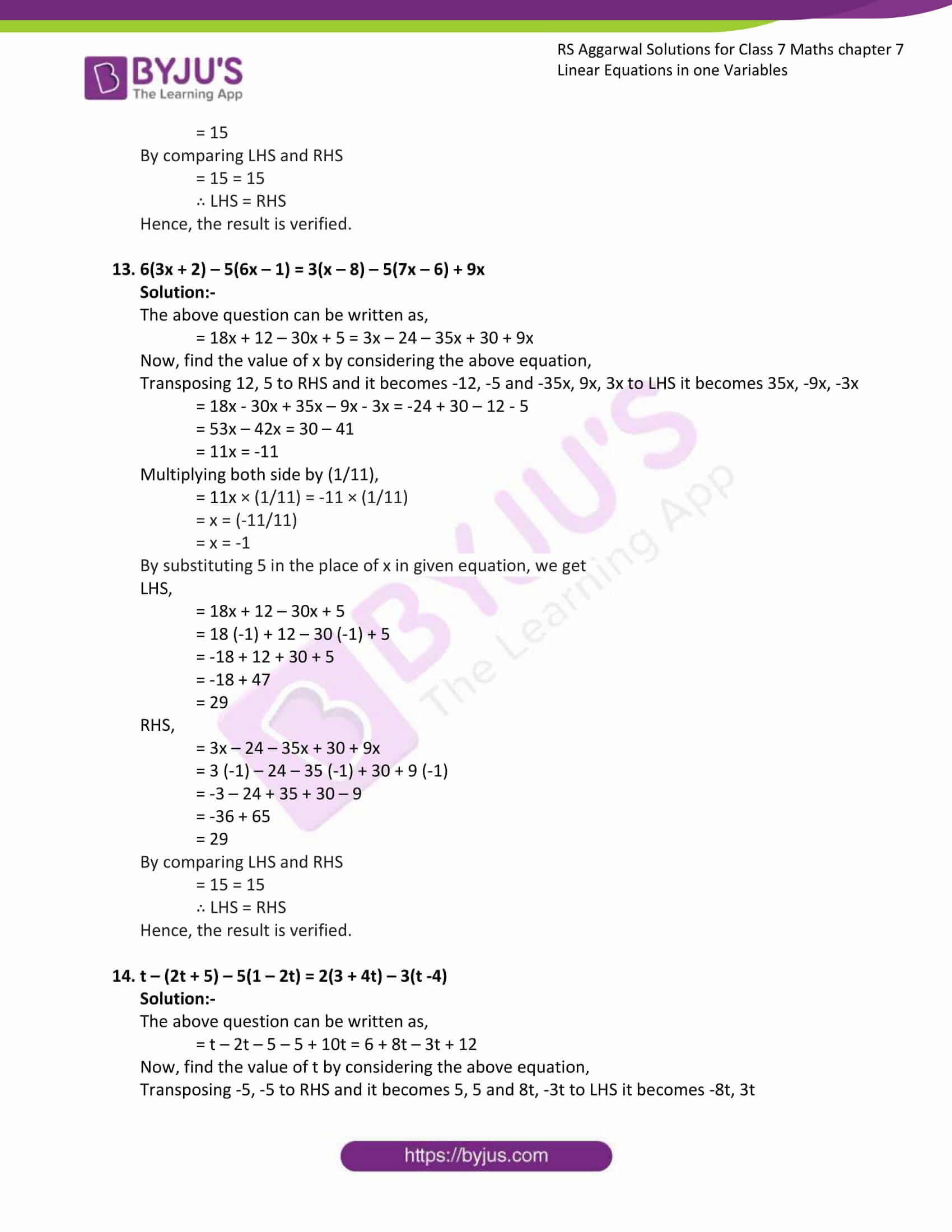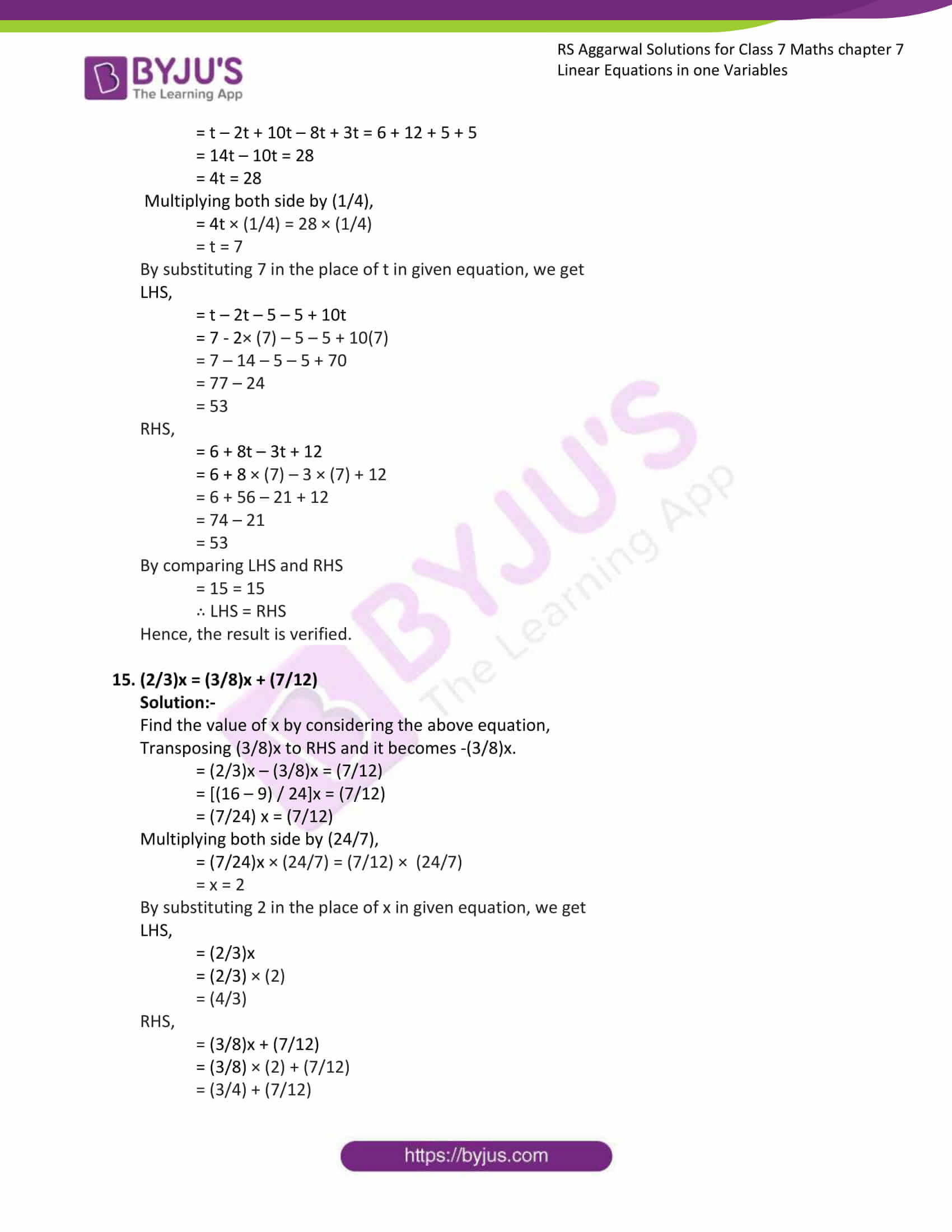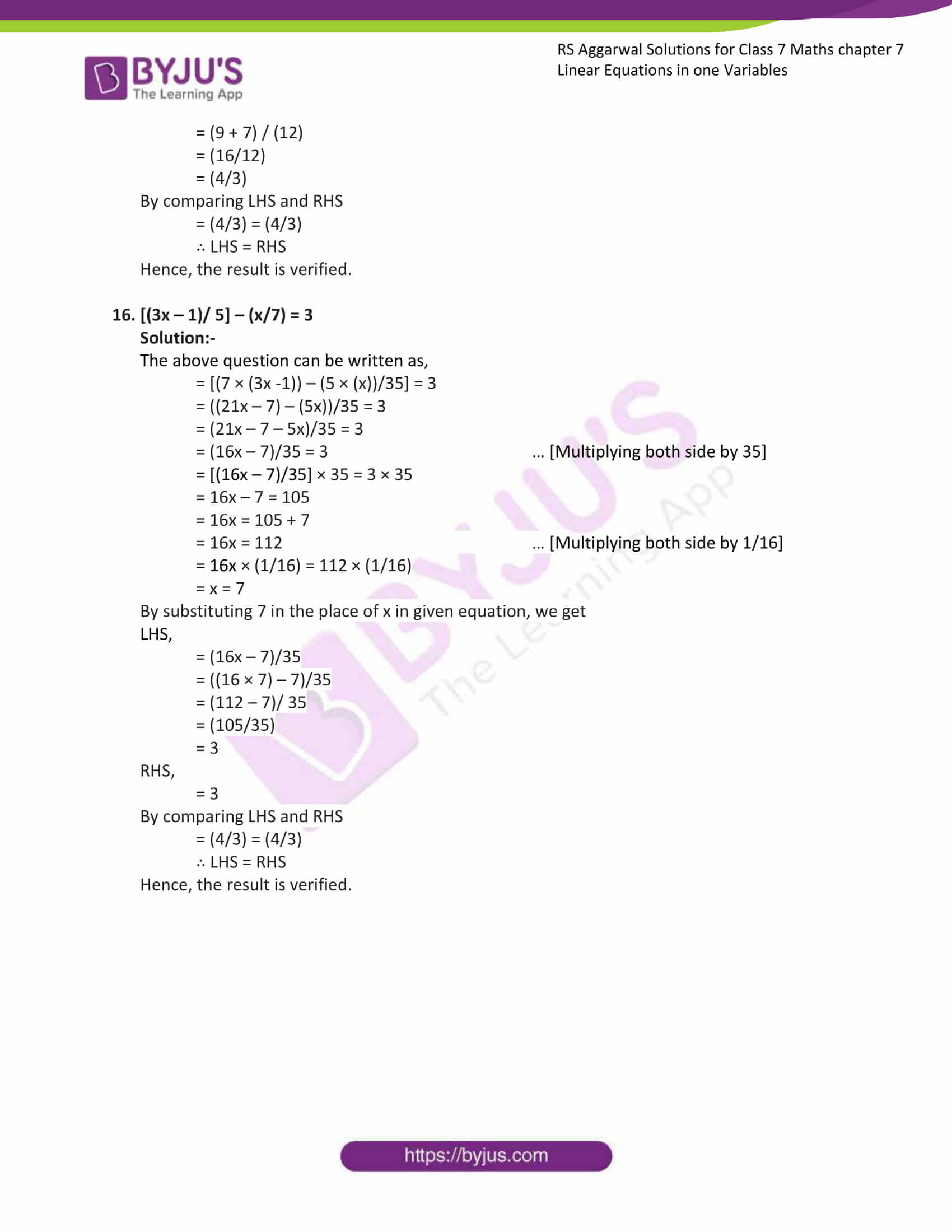### Access answers to Maths RS Aggarwal Solutions for Class 7 Chapter 7 – Linear Equations in One Variable Exercise 7A

Solve the following equations. Check your result in each case.

1. 3x – 5 = 0

Solution:-

The value of the variable which makes the equation a true statement is called the root of the equation.

Now, find the value of x by considering the given equation,

= 3x – 5 = 0

Transposing – 5 to RHS it becomes 5

= 3x = 5

Multiplying both side by (1/3)

= 3x × (1/3) = 5 × (1/3)

= x = (5/3)

By substituting (5/3) in the place of x in given equation, we get

= 3 × (5/3) – 5 = 0

= 5 – 5 = 0

= 0= 0

∴ LHS = RHS

Hence, the result is verified.

2. 8x – 3 = 9 – 2x

Solution:-

Now, find the value of x by considering the given equation,

=8x – 3 = 9 – 2x

Transposing – 3 to RHS and it becomes 3 and -2x to LHS it becomes 2x

= 8x + 2x = 9 + 3

= 10x = 11

Multiplying both side by (1/10)

= 10x × (1/10) = 12 × (1/10)

= x = (12/10)

= x = (6/5)

By substituting (6/5) in the place of x in given equation, we get

LHS,

= 8 × (6/5) – 3

= (48/5) – 3

= (48-15/5)

= (33/5)

RHS,

= 9 – 2 × (6/5)

= 9 – (12/5)

= (45 – 12)/5

= (33/5)

By comparing LHS and RHS

= (33/5) = (33/5)

∴ LHS = RHS

Hence, the result is verified.

3. 7 – 5x = 5 – 7x

Solution:-

Now, find the value of x by considering the given equation,

=7 – 5x = 5 – 7x

Transposing 7 to RHS and it becomes -7 and -7x to LHS it becomes 7x

= 7x – 5x = 5 – 7

= 2x = -2

Multiplying both side by (1/3)

= 2x × (1/2) = -2 × (1/2)

= x = (-2/2)

= x = -1

By substituting -1 in the place of x in given equation, we get

LHS,

= 7 – 5 × (-1)

= 7 – (-5)

= 7 + 5

= 12

RHS,

= 5 – 7 × (-1)

= 5 – (-7)

= 5 + 7

= 12

By comparing LHS and RHS

= 12 = 12

∴ LHS = RHS

Hence, the result is verified.

4. 3 + 2x = 1 – x

Solution:-

Now, find the value of x by considering the given equation,

=3 + 2x = 1 – x

Transposing 3 to RHS and it becomes -3 and -x to LHS it becomes x

= 2x + x = 1 – 3

= 3x = -2

Multiplying both side by (1/3)

= 3x × (1/3) = -2 × (1/3)

= x = (-2/3)

By substituting -2/3 in the place of x in given equation, we get

LHS,

= 3 + 2 × (-2/3)

= 3 + (-4/3)

= 3 – (4/3)

= (9 – 4) / 3

= 5/3

RHS,

= 1 – (-2/3)

= 1 + (2/3)

= (3 + 2)/ 3

= 5/3

By comparing LHS and RHS

= 5/3 = 5/3

∴ LHS = RHS

Hence, the result is verified.

5. 2 (x – 2) + 3 (4x – 1) = 0

Solution:-

The above question can be written as,

= 2x – 4 + 12x – 3 = 0

Now, find the value of x by considering the above equation,

= 2x + 12x – 4 – 3 = 0

= 14x – 7 = 0

Transposing -7 to RHS and it becomes 7

= 14x = 7

Multiplying both side by (1/14)

= 14x × (1/14) = 7 × (1/14)

= x = (7/14)

= x = 1/2

By substituting 1/2 in the place of x in given equation, we get

LHS,

= (2 × (1/2)) – 4 + (12 × (1/2)) – 3

= 1– 4 + 6 – 3

= 7 – 7

= 0

By comparing LHS and RHS

= 0 = 0

∴ LHS = RHS

Hence, the result is verified.

6. 5 (2x – 3) – 3 (3x – 7) = 5

Solution:-

The above question can be written as,

= 10x – 15 – 9x + 21 = 5

Now, find the value of x by considering the given equation,

= 10x – 15 – 9x + 21 = 5

= x + 6 = 5

Transposing 6 to RHS and it becomes -6

= x = 5 – 6

= x = -1

By substituting -1 in the place of x in given equation, we get

LHS,

= 10 (-1) -15 -9(-1) + 21

= -10 – 15 + 9 + 21

= -25 + 30

= 5

By comparing LHS and RHS

= 5 = 5

∴ LHS = RHS

Hence, the result is verified.

7. 2x – (1/3) = (1/5) – x

Solution:-

Now, find the value of x by considering the given equation,

=2x – (1/3) = (1/5) – x

Transposing – (1/3) to RHS and it becomes (1/3) and – x to LHS it becomes x

= 2x + x = (1/5) + (1/3)

= 3x = (3 + 5) / 15

= 3x = 8/15

Multiplying both side by (1/3)

= 3x × (1/3) = (8/15) × (1/3)

= x = (8/45)

By substituting (8/45) in the place of x in given equation, we get

LHS,

= 2 × (8/45) – (1/3)

= (16/45) – (1/3)

= (16 – 15) / 45

= (1/45)

RHS

= (1/5) – (8/45)

= (9-8) / 45

= (1/45)

By comparing LHS and RHS

= (1/45) = (1/45)

∴ LHS = RHS

Hence, the result is verified.

8. (1/2)x- 3 = 5 + (1/3)x

Solution:-

Now, find the value of x by considering the given equation,

= (1/2)x- 3 = 5 + (1/3)x

Transposing -3 to RHS and it becomes 3 and (1/3)x to LHS it becomes (-1/3)x

= (1/2)x – (1/3)x = 5 + 3

= ((3-2)/6)x = 8

= (1/6)x = 8

Multiplying both side by 6

= 6 × (1/6)x = 8 × 6

= x = 48

By substituting 48 in the place of x in given equation, we get

LHS,

= (1/2) × (48) – 3

= (48/2) – 3

= 24 – 3

= 21

RHS,

= 5 + (1/3) × (48)

= 5 + (48/3)

= 5 + 16

= 21

By comparing LHS and RHS

= 21 = 21

∴ LHS = RHS

Hence, the result is verified.

9. (x/2) + (x/4) = (1/8)

Solution:-

Now, find the value of x by considering the given equation,

= (x/2) + (x/4) = (1/8)

= ((2 + 1) / 4) = (1/8)

= (3/4)x = (1/8)

Multiplying both side by (4/3)

= (3/4)x × (4/3) = (1/8) × (4/3)

= x = (1/6)

By substituting (1/6) in the place of x in given equation, we get

LHS,

= (3/4)x

= (3/4) × (1/6)

= (1/8)

RHS,

= (1/8)

By comparing LHS and RHS

= (1/8) = (1/8)

∴ LHS = RHS

Hence, the result is verified.

10. 3x + 2(x + 2) = 20 – (2x – 5)

Solution:-

The above question can be written as,

= 3x + 2x + 4 = 20 – 2x + 5

Now, find the value of x by considering the above equation,

= 5x + 4 = 20 – 2x + 5

Transposing 4 to RHS and it becomes -4 and -2x to LHS it becomes 2x

= 5x + 2x = 20 – 4 + 5

= 7x = 25 – 4

= 7x = 21

Multiplying both side by (1/7)

= 7x × (1/7) = 21 × (1/7)

= x = 21/7

= x = 3

By substituting 3 in the place of x in given equation, we get

LHS,

= 5x + 4

= 5 × (3) + 4

= 15 + 4

= 19

RHS,

= 20 – 2x + 5

= 20 – 2 × (3) + 5

= 20 – 6 + 5

= 25 – 6

= 19

By comparing LHS and RHS

= 19 = 19

∴ LHS = RHS

Hence, the result is verified.

11. 13( y – 4) – 3(y-9) – 5(y + 4) = 0

Solution:-

The above question can be written as,

= 13y – 52 – 3y + 27 – 5y – 20 = 0

Now, find the value of y by considering the above equation,

= 13y – 3y – 5y – 52 + 27 – 20 = 0

= 13y – 8y – 72 + 27 = 0

= 5y – 45 = 0

Transposing -45 to RHS and it becomes 45

= 5y = 45

Multiplying both side by (1/5)

= 5y × (1/5) = 45 × (1/5)

= y = 9

By substituting 9 in the place of y in given equation, we get

LHS,

= 5y – 45

= 5 × (9) – 45

= 45 – 45

= 0

RHS,

= 0

By comparing LHS and RHS

= 0 = 0

∴ LHS = RHS

Hence, the result is verified.

12. (2m + 5) / 3 = (3m – 10)

Solution:-

The above question can be written as,

= 3 × [(2m + 5)/ 3] = 3 × (3m -10) … [Multiplying both side by 3]

= (2m + 5) = 9m – 30

Transposing 2m to RHS and it becomes -2m and -30 to LHS it becomes 30

= 30 + 5 = 9m -2m

= 35 = 7m

Multiplying both side by (1/7),

= 7m × (1/7) = 35 × (1/7)

= m = (35/7)

= m = 5

By substituting 5 in the place of m in given equation, we get

LHS,

= 2m + 5

= 2 × (5) + 5

= 10 + 5

= 15

RHS,

= 9m – 30

= 9 × (5) – 30

= 45 – 30

= 15

By comparing LHS and RHS

= 15 = 15

∴ LHS = RHS

Hence, the result is verified.

13. 6(3x + 2) – 5(6x – 1) = 3(x – 8) – 5(7x – 6) + 9x

Solution:-

The above question can be written as,

= 18x + 12 – 30x + 5 = 3x – 24 – 35x + 30 + 9x

Now, find the value of x by considering the above equation,

Transposing 12, 5 to RHS and it becomes -12, -5 and -35x, 9x, 3x to LHS it becomes 35x, -9x, -3x

= 18x – 30x + 35x – 9x – 3x = -24 + 30 – 12 – 5

= 53x – 42x = 30 – 41

= 11x = -11

Multiplying both side by (1/11),

= 11x × (1/11) = -11 × (1/11)

= x = (-11/11)

= x = -1

By substituting 5 in the place of x in given equation, we get

LHS,

= 18x + 12 – 30x + 5

= 18 (-1) + 12 – 30 (-1) + 5

= -18 + 12 + 30 + 5

= -18 + 47

= 29

RHS,

= 3x – 24 – 35x + 30 + 9x

= 3 (-1) – 24 – 35 (-1) + 30 + 9 (-1)

= -3 – 24 + 35 + 30 – 9

= -36 + 65

= 29

By comparing LHS and RHS

= 15 = 15

∴ LHS = RHS

Hence, the result is verified.

14. t – (2t + 5) – 5(1 – 2t) = 2(3 + 4t) – 3(t -4)

Solution:-

The above question can be written as,

= t – 2t – 5 – 5 + 10t = 6 + 8t – 3t + 12

Now, find the value of t by considering the above equation,

Transposing -5, -5 to RHS and it becomes 5, 5 and 8t, -3t to LHS it becomes -8t, 3t

= t – 2t + 10t – 8t + 3t = 6 + 12 + 5 + 5

= 14t – 10t = 28

= 4t = 28

Multiplying both side by (1/4),

= 4t × (1/4) = 28 × (1/4)

= t = 7

By substituting 7 in the place of t in given equation, we get

LHS,

= t – 2t – 5 – 5 + 10t

= 7 – 2× (7) – 5 – 5 + 10(7)

= 7 – 14 – 5 – 5 + 70

= 77 – 24

= 53

RHS,

= 6 + 8t – 3t + 12

= 6 + 8 × (7) – 3 × (7) + 12

= 6 + 56 – 21 + 12

= 74 – 21

= 53

By comparing LHS and RHS

= 15 = 15

∴ LHS = RHS

Hence, the result is verified.

15. (2/3)x = (3/8)x + (7/12)

Solution:-

Find the value of x by considering the above equation,

Transposing (3/8)x to RHS and it becomes -(3/8)x.

= (2/3)x – (3/8)x = (7/12)

= [(16 – 9) / 24]x = (7/12)

= (7/24) x = (7/12)

= x = 2

By substituting 2 in the place of x in given equation, we get

LHS,

= (2/3)x

= (2/3) × (2)

= (4/3)

RHS,

= (3/8)x + (7/12)

= (3/8) × (2) + (7/12)

= (3/4) + (7/12)

= (9 + 7) / (12)

= (16/12)

= (4/3)

By comparing LHS and RHS

= (4/3) = (4/3)

∴ LHS = RHS

Hence, the result is verified.

16. [(3x – 1)/ 5] – (x/7) = 3

Solution:-

The above question can be written as,

= [(7 × (3x -1)) – (5 × (x))/35] = 3

= ((21x – 7) – (5x))/35 = 3

= (21x – 7 – 5x)/35 = 3

= (16x – 7)/35 = 3 … [Multiplying both side by 35]

= [(16x – 7)/35] × 35 = 3 × 35

= 16x – 7 = 105

= 16x = 105 + 7

= 16x = 112 … [Multiplying both side by 1/16]

= 16x × (1/16) = 112 × (1/16)

= x = 7

By substituting 7 in the place of x in given equation, we get

LHS,

= (16x – 7)/35

= ((16 × 7) – 7)/35

= (112 – 7)/ 35

= (105/35)

= 3

RHS,

= 3

By comparing LHS and RHS

= (4/3) = (4/3)

∴ LHS = RHS

Hence, the result is verified.

### Access other exercises of RS Aggarwal Solutions For Class 7 Chapter 7 – Linear Equations in One Variable

Exercise 7C Solutions